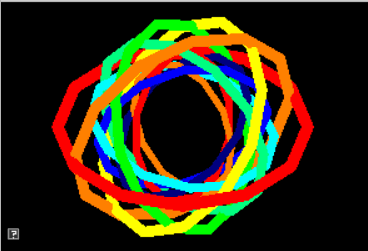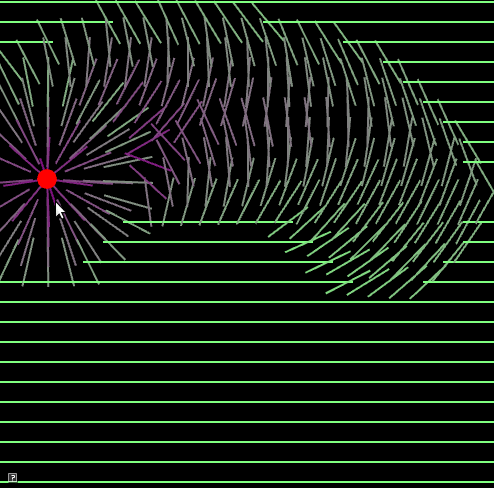## Assignment 5: Quazy Quaternions

Quaternions, matrices, and you!

Due Friday, Oct 22, before midnight

The goals of this lab are to

• Implement angle/axis to quaternion conversions

• Implement quaternion to matrix conversions

• Use rotations in an animation (based on the code your wrote for lab 4)

After completing this assignment, all teapots from lab 4 should spin in unison!

## Get the source

On Github, do a `Fetch Upstream` to synchronize your forked repository with the class repository.

Then update the source on your local machine and rebuild.

``````> git pull
> cd build
> cmake ..; make``````

## 1. Quaternion

Finish the quaternion implementation in `libsrc/atkmath`. Use the utility, `a5-test-quat` to check your work.

``build> ../bin/a5-test-quat``

Once all your conversions are working, your teapot demo will also be fully operational!

### 1.1. Angle/Axis

Implement conversions between quaternions and the angle-axis representations for rotation.

Recall that the relationship between quaternions and an angle/axis rotation is

$q = \left[\sin(\frac{\theta}{2}) \hat{u}, \cos(\frac{\theta}{2})\right]$

• Implement `Quaternion::fromAngleAxis` in `libsrc/atkmath/Quaternion-basecode.cpp`

• Implement `Quaternion::toAngleAxis` in `libsrc/atkmath/Quaternion-basecode.cpp`

• Implement `Matrix3::fromAngleAxis` in `libsrc/atkmath/Matrix3-basecode.cpp` using a direct angle/axis to matrix implementation

• Implement `Matrix3::toAngleAxis` in `libsrc/atkmath/Matrix3-basecode.cpp` using a direct matrix to angle/axis implementation

To run the unit tests from the <b>build</b> directory, type

``build> ../bin/a5-test-quat``

Accessing member variables in Quaternion works similarly to Matrix3

``````void Quaternion::foo() {
// accessing member variables in Quaternion works similarly to Matrix3
mX = 0.5;
mY = 0.0;
mZ = 3.0;
mW = -5.0;
normalize(); // make myself a unit quaternion
}

void foo() {
AQuaternion q; // create an identity quat
q = 0.1; // x value
q = 0.1; // y value
q = 0.1; // z value
q = 0.1; // w value
q.normalize(); // make q a unit quaternion
}``````

The output of `test-quat` should be

``````45 Z 0 0 0.382683 0.92388 0 0 1 45
45 Y 0 0.382683 0 0.92388 0 1 0 45
45 X 0.382683 0 0 0.92388 1 0 0 45``````

### 1.2. Matrix

In class, we derived the rotation matrix corresponding to a quaternion

$q = (x, y, z, w) = (\bar{v}, w )$
$\left( \begin{array}{ccc} 1-2(y^2+z^2) & 2(x y - w z) & 2(x z + w y) \\ 2(x y + w z) & 1-2(x^2+z^2) & 2(y z - w x) \\ 2(x z - w y) & 2(y z + w x) & 1-2(y^2+x^2) \\ \end{array} \right)$
• Implement the above matrix to convert from a Quaternion to a Matrix3 in `Quaternion::toMatrix()` located in `libsrc/atkmath/Quaternion-basecode.cpp`

• Implement the algorithm for converting from Matrix3 to Quaternion in `Quaternion::fromMatrix()` in `libsrc/atkmath/Quaternion-basecode.cpp`.

To run the tests from the `build` directory, type

``````build> ../bin/a5-test-quat-mat
build> ../bin/a4-teapots``````

The output of `test-quat-mat` should be

``````45 Z 0 0 0.382683 0.92388
45 Z
0.707107 -0.707107 0
0.707107 0.707107 0
0 0 1

45 Y 0 0.382683 0 0.92388
45 Y
0.707107 0 0.707107
0 1 0
-0.707107 0 0.707107

45 X 0.382683 0 0 0.92388
45 X
1 0 0
0 0.707107 -0.707107
0 0.707107 0.707107``````

## 2. Be Unique!

Implement your own unique animation or image in `a5-quat/unique.cpp`. Some ideas:

• Make a new demo with objects that rotate around an arbitrary axis

• Create a shape whose orientation and position is controlled using the keyboard### 3.1. What to hand-in

• Images, movies, gifs, as appropriate

### 3.2. How to hand-in

Check-in your code, images, and writeup and push the changes to Github. If everything is uploaded correctly, you will see your work on Github.

``````> cd animation-toolkit
> git commit -m "helpful message"
> git push``````

Best practice is to always commit changes as you work, rather than waiting until the end to commit changes. You can always revert to an old version if you need to!

### 3.3. Generating images, movies and gifs

Screenshots

On Ubuntu, you can take a screenshot by

• Prt Scrn to take a screenshot of the desktop.

• Alt + Prt Scrn to take a screenshot of a window.

Gifs

On Ubuntu, you can use Peek to create gifs.

Movies

On Ubuntu, you can use `recordmydesktop` to record movies in .ogv format.

By default, the whole desktop is recorded unless you give it a window id. To get the window id, call `xwininfo` and click on the window. Then pass the id to `recordmydesktop`.

``> recordmydesktop --windowid <WindowId> --no-sound  --v_quality 30 -o <name>.ogv``

To check the video, open it in firefox.

``> firefox <name>.ogv``
 Files larger than 100 MB cannot be checked into git. In general, videos should be less than 5 MB.

Update `assignments/a5-quat/Readme.md` to document the features in your assignment. Your readme should contain your images, gifs, and movies.
``![Alternate text here](/path/to/image.png)``